Triangular Numbers

How to Find Triangular Numbers

A triangular number (also known as triangle number) include objects organized in an equilateral triangle. The nth triangular number is the number of black dots in the triangular pattern with n black dots on a side and is equivalent to the total of the "n" natural numbers from "1" to "n". The arrangement of triangular numbers, beginning at the 0th triangular number, is:
0, 1, 3, 6, 10, 15, 21, 28, 36, 45, 55, 66, 78, 91, 105, 120, 136, 153, 171, 190, 210, 231, 253, 276, 300, 325, 351, 378, 406, 435, 465, 496, 528, 561, 595, 630,

The triangle numbers can be calculated by the formulas given below: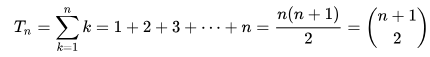Where (n+1)/2 is the Binomial coefficient. It shows the number of distinct pairs that can be chosen from (n + 1) objects, and it is said as "n plus one to one choose two".

The first equation can be represented using an image. For every triangular number Tn, think of a "half-square" pattern of objects corresponding to the triangular number, as shown in the image below. Replicating this pattern and turning it upside down to create a rectangular image doubles the number of objects, giving a rectangle with dimensions n×(n+1), which is also the number of objects in the rectangle. It clearly shows that the triangular number itself is in every case precisely half of the number of objects in such an image or Tn= (n (n+1)/2).

For example, for T4(n=4),
2T4= 4(4+1) = 20 (green & yellow)
⇒ T4= (4(4+1))/2 = 10 green dots.

The first equation can also be derived with the help of mathematical induction. setting the inductive hypothesis for let's say n and adding n+1 to both sides gives: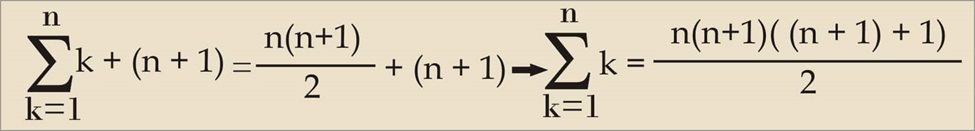We can also say, since the proposition P(n) (which is, the first equation, or inductive hypothesis) is true when n=1, and since P(n) being true implies that P(n+1) is also true, then the previous equation stands true for all-natural numbers. The above argument can be modified to start with and include zero.

Carl Friedrich Gauss found this relationship by multiplying n/2pairs of numbers in the sum by the values of each pair n + 1.

However, Gauss was not the first to discover this formula, and some people say that its origin goes back to the Pythagoreans 5th century BC. The two formulas were described by the Irish monk Dicuil in his Computes.

The triangular number Tn also solves the handshake problem of counting the number of handshakes that will happen if each person in a room with n + 1 people shakes hands once with each person. This means that the solution to the handshake problem of n people is Tn−1. The function T is the additive analog of the factorial function, which is the product of integers from "1" to "n".

The line segments between closest pairs of dots in the triangle can be presented in terms of the number of dots or with a recurrence relation: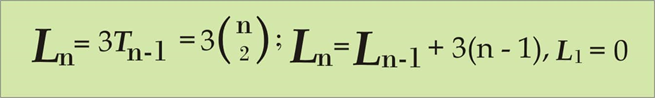In the limit, the ratio of the two numbers, dots and line segments is: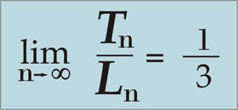Relation with other figurate numbers:

Triangle numbers do have a wide variety of relations to other figurate numbers. The sum of two consecutive triangular numbers is a square value. Algebraically,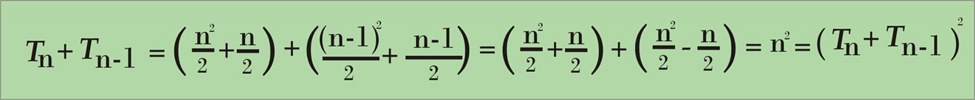This fact can be represented graphically by placing the triangles in opposite directions to create a square:

There are infinite numbers of triangular numbers that are also square value such as 1, 36, 1225, etc.
Some of them can be calculated by using a simple recursive formula: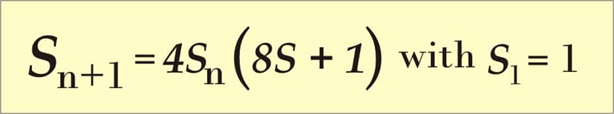Note: All square triangular numbers are generated from the recursion.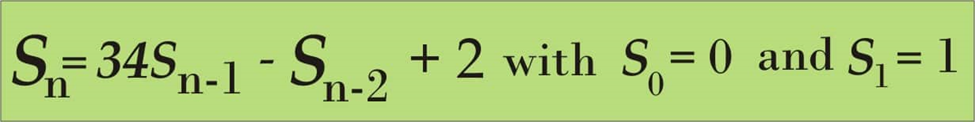Also, the square value of the nth triangle number is the same as the sum of the cubes of the integers from "1" to "n". This can also be represented as: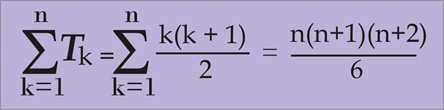The difference between the nth m-gon number and the nth (m + 1)-gon number is the (n − 1) th triangle number. For example, the difference between the sixth heptagonal number and the sixth hexagonal number is the fifth triangular number. Every other triangle number is a hexagonal number. Knowing the triangle numbers, one can calculate any centered polygonal number; the nth centered k-gon number is obtained by the formula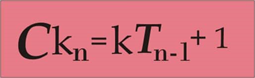Where T is a triangle number.

The difference between the two triangular numbers is a trapezoidal number.

Other properties

• • Triangular numbers correlate to the first-degree case of Faulhaber’s formula.

• • It’s interesting to notice that every alternate triangular number (1, 6, 15, 28, 45….) are also hexagonal numbers.

• • Every even perfect number is triangular (as well as hexagonal), according to the given formula.

•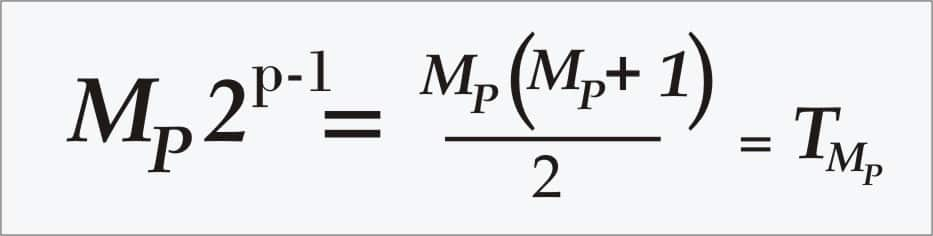Here, ‘Mp’ is a Mersenne prime. No odd perfect numbers are known, therefore, all known perfect numbers are triangular.
For example, the third triangle number is (3 × 2 =) 6, the seventh is (7 × 4 =) 28, the 31st is (31 × 16 =) 496, and the 127th is (127 × 64 =) 8128.

• • In the case of base 10, the digital root of a nonzero triangular number is always 1, 3, 6, or 9. Hence every triangular number is either divisible by 3 or it leaves remainder of 1 when divided by 9:

• 0 = 9 × 0
1 = 9 × 0 + 1
3 = 9 × 0 + 3
6 = 9 × 0 + 6
10 = 9 × 1 + 1
15 = 9 × 1 + 6
21 = 9 × 2 + 3
28 = 9 × 3 + 1
36 = 9 × 4
45 = 9 × 5
55 = 9 × 6 + 1
66 = 9 × 7 + 3
78 = 9 × 8 + 6
91 = 9 × 10 + 1

• • Triangular numbers have one more specific property that they aren't divisible by 3, that is, when divided by 27, they either have leave a remainder of 1 or 10. Those equal to 10 mod 27, are equal to 10 mod 81 too.

• • The digital root pattern for triangular numbers are repeating every nine terms, as shown in the table above, is "1, 3, 6, 1, 6, 3, 1, 9, 9".

• • The converse of the statement above is, not necessarily’ always holds true. For example, the digital root of 12(not a triangular number), is 3 and divisible by three.

• Suppose, x is a triangular number, then ax + b is also a triangular number, given a, which is an odd-square and b = (a – 1)/8.
Where, b will always be a triangular number because Tn + 1 = (2n + 1)2/8, which gives all the odd squares are revealed by multiplication of 8 to the triangle number and adding 1, and the process for b given a is an odd square is the inverse of this operation.

The first few pairs of this form (excluding 1x + 0) are: 9x + 1, 25x + 3, 49x + 6, 81x + 10, 121x + 15, 169x + 21 … and so on. Provided, x is equal to Tn, these formulas yield T3n + 1, T5n + 2, T7n + 3, T9n + 4, so on and so forth.

The summation of the reciprocals of all the non-zero triangle numbers is: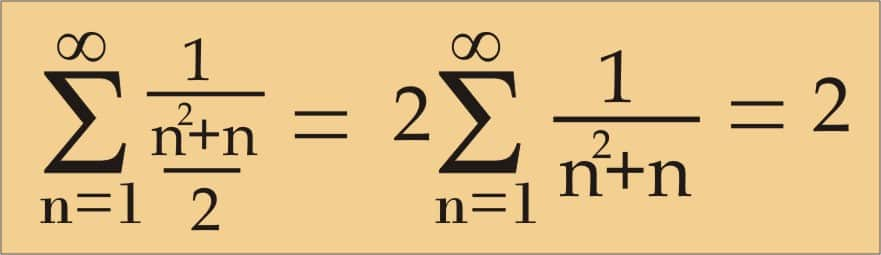This can be shown through a sum of telescopic series: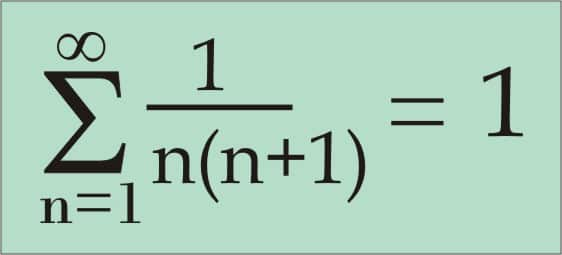Two other formulas relating to triangle numbers are: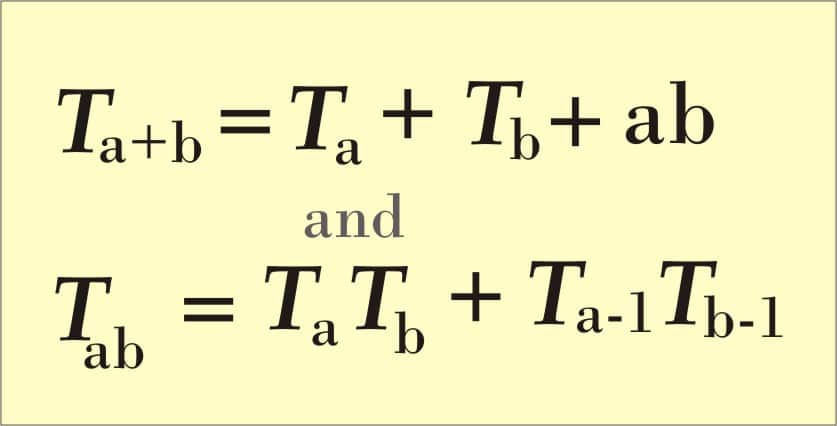Both of these can be established either by looking at dot patterns (shown above) or with some simple algebra.
In 1796, a German mathematician and scientist Carl Fredrich Gauss discovered that every positive integer can be represented as a sum of three triangular numbers (possibly including T0= 0), num = Δ + Δ + Δ".

Note: This theorem does not say that the triangular numbers are different (as in the case of 20 = 10 + 10 + 0), nor does it say that a solution with exactly three non-zero triangle numbers has to exist: a special case of the Fermat Polygonal number Theorem.

The largest triangular number of the form 2k − 1 is 4095(reference Ramanujan Nagell equation).
Wacław Franciszek Sierpiński raised the question as to the existence of four distinct triangular numbers in geometric progression. It was concluded by Polish mathematician Kazimierz Szymiczek that it was impossible and was later proven by Fang and Chen also in 2007.

Applications

A fully connected network of n computing devices needs the presence of Tn − 1 cables or other connections; this is equivalent to the handshake problem mentioned above.

In a tournament format that uses a round-robin group stage, the number of matches that are required to be played between n teams is equal to the triangular number Tn − 1. For example, a group stage with 4 teams needs 6 matches, and a group stage with 8 teams requires 28 matches. This is also similar to the handshake problem and fully connected network problems.

One way of calculating the depreciation of an asset is the sum of years' digit method, which involves finding Tn, where n is the length in years of the asset's useful life. Every year, the item loses (b − s) × n − y/ Tn, where b is the item's starting value (in units of currency), ‘s’ is its final salvage values’’ is the total number of years for which the item is usable, and ‘y’ the current year in the depreciation schedule. In this method, an item with a usable life of n = 4 years would lose 4/10 of its "losable" value in the first year, 3/10 in the second, 2/10in the third, and 1/10 in the fourth, accumulating a total depreciation of 10/10 (the whole) of the losable value.

Triangular roots and tests for triangular numbers
Through analogy with the square root of x, (positive) triangular root of x can be defined as the number n such that Tn = x: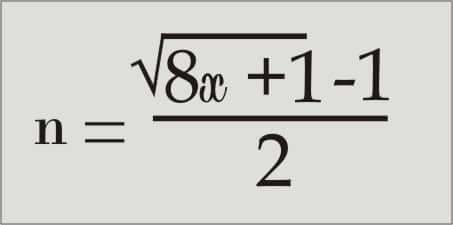This follows immediately from the quadratic formula. So, an integer x will be triangular if and only if 8x + 1 is a square. Similarly, if the positive triangular root n of x is an integer, then x is the nth triangular number.